# A firm with greater operating leverage would have a higher WACC. (all else equal). True or...

A firm with greater operating leverage would have a higher WACC. (all else equal).

True or False?

The weighted average cost of capital is affected by the financial leverage that is when the company changes the capital structure of the firm while the operating leverage is a measure of change in earning before interest and tax in comparison to change in sales .

##### Add Answer of: A firm with greater operating leverage would have a higher WACC. (all else equal). True or...
Similar Homework Help Questions
• ### all else being equal, a company with a high operating leverage will have All else being equal, a company with a hig...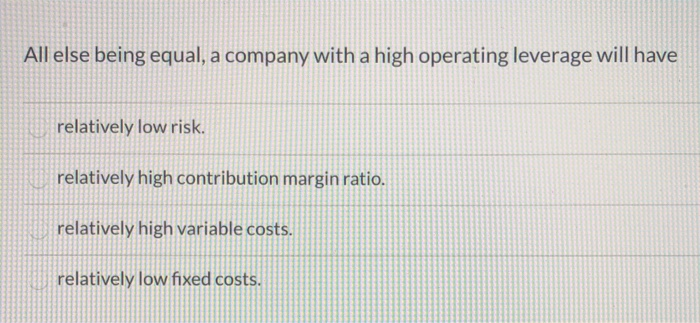all else being equal, a company with a high operating leverage will have All else being equal, a company with a high operating leverage will have relatively low risk. relatively high contribution margin ratio. relatively high variable costs. relatively low fixed costs.

• ### Question 10 Which of the following is true about the degree of operating leverage? Operating leverage...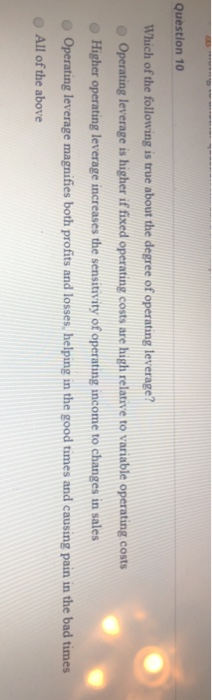Question 10 Which of the following is true about the degree of operating leverage? Operating leverage is higher if fixed operating costs are high relative to variable operating costs Higher operating leverage increases the sensitivity of operating income to changes in sales Operating leverage magnifies both profits and losses, helping in the good times and causing pain in the bad times All of the above

• ### Two hypothetical firms have the same net income and the same total assets. One firm has...

Two hypothetical firms have the same net income and the same total assets. One firm has much higher Return on Equity (ROE). It must be true that: The firm with the higher ROE must have less leverage in the capital structure The firm with the higher ROE must have more leverage in the capital structure All else equal, differences in leverage will not affect ROE Increased leverage can only decrease risk, but not increase returns None of the above

• ### 2. What is operating leverage? How, if at all, is it similar to financial leverage? If...

2. What is operating leverage? How, if at all, is it similar to financial leverage? If a firm has high operating leverage would you expect it to have high or low financial leverage? Explain your reasoning. Please also add to your answer an example of where an HR manager may use operating leverage to his or her advantage.

• ### Which of the following is true about the concept of leverage? O A. at the breakeven point, operating leverage is equal...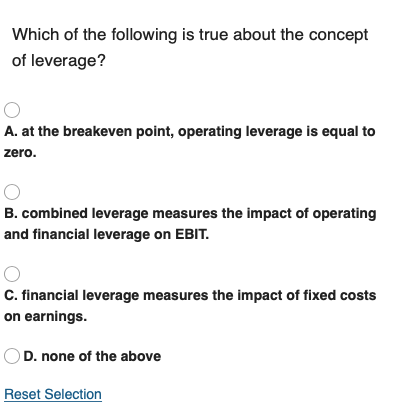Which of the following is true about the concept of leverage? O A. at the breakeven point, operating leverage is equal to zero. O B. combined leverage measures the impact of operating and financial leverage on EBIT. O C. financial leverage measures the impact of fixed costs on earnings. OD. none of the above Reset Selection

• ### 5. Highly leveraged firms have higher ROE than lower leveraged firms. 6. All things equal, the...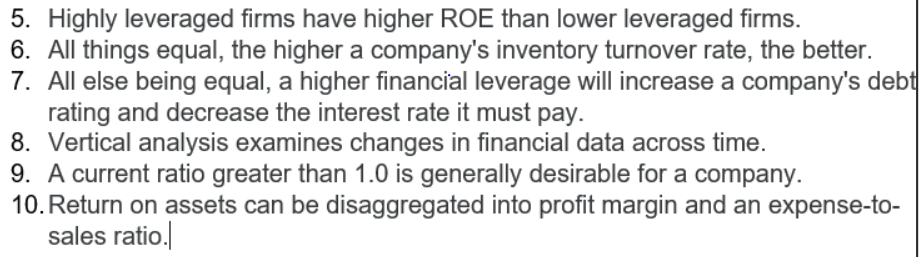5. Highly leveraged firms have higher ROE than lower leveraged firms. 6. All things equal, the higher a company's inventory turnover rate, the better. 7. All else being equal, a higher financial leverage will increase a company's debt rating and decrease the interest rate it must pay. 8. Vertical analysis examines changes in financial data across time. 9. A current ratio greater than 1.0 is generally desirable for a company. 10. Return on assets can be disaggregated into profit margin...

• ### the higher the interest rate is the higher the duration, all else being equal t/f?

the higher the interest rate is the higher the duration, all else being equal t/f?

• ### All else being equal, high expense ratio negatively affects returns to fund investors True or False...

All else being equal, high expense ratio negatively affects returns to fund investors True or False True False

• ### All else being equal, diverse teams generally outperform non-diverse teams. True False

All else being equal, diverse teams generally outperform non-diverse teams. True False

• ### pls answer all Which stock should have the higher price? ALL ELSE EQUAL Stock A has...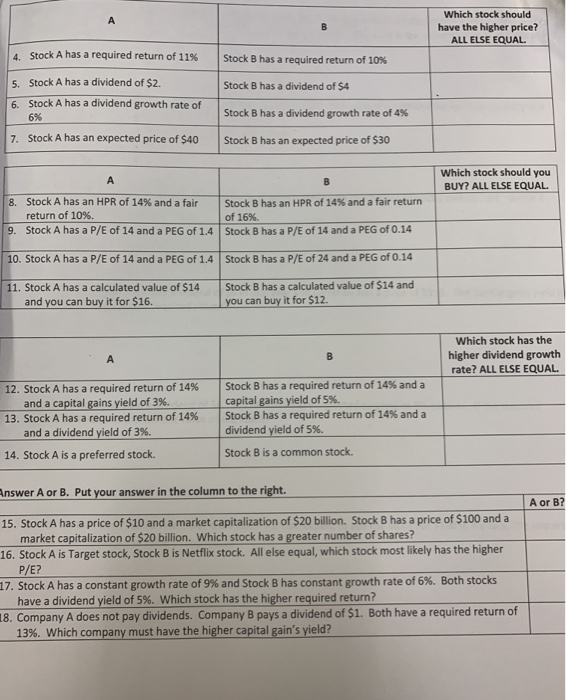pls answer all Which stock should have the higher price? ALL ELSE EQUAL Stock A has a required return of 11% Stock Bhas a required return of 10% 5. Stock A has a dividend of \$2. 6. Stock A has a dividend growth rate of 696 Stock B has a dividend of 54 Stock B has a dividend growth rate of 4% 7. Stock A has an expected price of \$40 Stock Bhas an expected price of \$30 Which stock...

Free Homework App